# Models

The following models are currently implemented in OpenCMP. In general, all models are expected to include a standard Galerkin finite element method formulation, a discontinuous Galerkin formulation, and a diffuse interface formulation. Nonlinear models are further expected to include formulations for Oseen-style linearization and IMEX linearization. The following sections provide the weak forms for all implemented formulations of the available models.

## Poisson Equation

The Poisson equation takes the following form on a simulation domain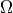. There are three possible types of boundary condition - Dirichlet, Neumann, and Robin - applied on boundary regions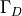,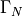, and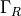respectively.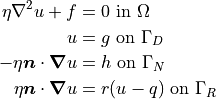where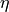is a constant typically taken to be the diffusivity and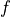is some source function.

The different formulations of the Poisson equation finite element weak form are given below. Note that in all cases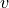is the trial function. In the case of the discontinuous Galerkin method,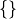and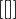refer to the average and jump operators respectively and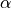is the penalty coefficient. In the case of the diffuse interface method,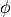is the phase field,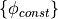are masks for different boundary condition regions, and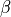is the Nitsche method penalty parameter. Also note that for the diffuse interface method all integrals become volume integrals over the enclosing simple domain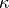.

### Standard Galerkin Finite Element Formulation

The standard Galerkin finite element method formulation is as follows: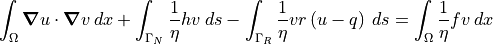Dirichlet boundary conditions are imposed strongly by setting values at applicable boundary degrees of freedom.

### Discontinuous Galerkin Formulation

The discontinuous Galerkin formulation is as follows: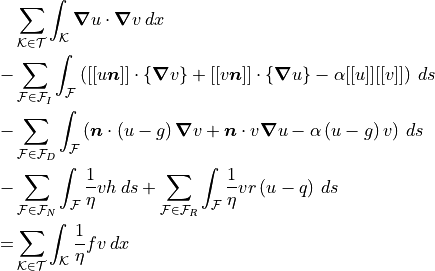where volume integrals are over individual mesh elementsthen summed over the entire triangulation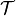. Surface integrals are over mesh element facets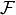and summed over either interior facets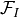or boundary facets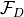,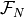, and.

### Diffuse Interface Formulation

The diffuse interface formulation is as follows for the standard Galerkin finite element method: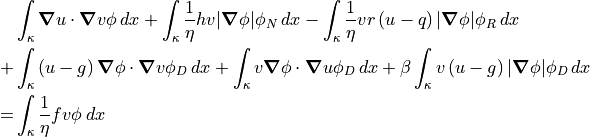and for the discontinuous Galerkin method: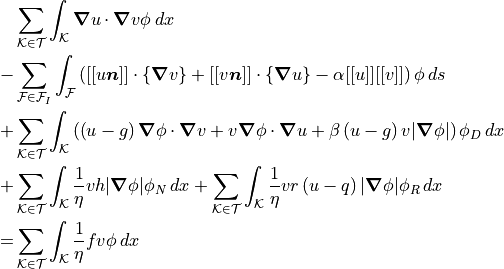## Stokes Equations

The Stokes equations take the following form on a simulation domain. There are two possible types of boundary condition - velocity Dirichlet and normal stress - applied on boundary regionsand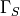respectively.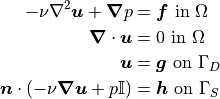where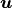is the velocity and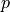is the pressure. Furthermore,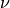is the constant kinematic viscosity andis some body force.

The different formulations of the Stokes equations finite element weak form are given below. Note that in all cases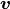and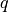are the trial functions for velocity and pressure respectively. In the case of the discontinuous Galerkin method,andrefer to the average and jump operators respectively andis the penalty coefficient. In the case of the diffuse interface method,is the phase field,are masks for different boundary condition regions, andis the Nitsche method penalty parameter. Also note that for the diffuse interface method all integrals become volume integrals over the enclosing simple domain.

### Standard Galerkin Finite Element Formulation

The standard Galerkin finite element method formulation is as follows: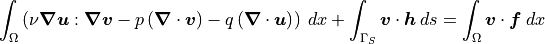Dirichlet boundary conditions are imposed strongly by setting values at applicable boundary degrees of freedom.

### Discontinuous Galerkin Formulation

The discontinuous Galerkin formulation is as follows: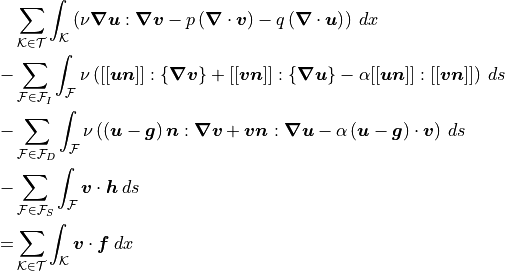where volume integrals are over individual mesh elementsthen summed over the entire triangulation. Surface integrals are over mesh element facetsand summed over either interior facetsor boundary facetsand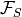.

### Diffuse Interface Formulation

The diffuse interface formulation is as follows for the standard Galerkin finite element method: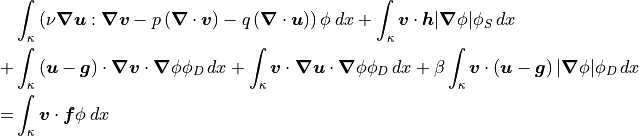and for the discontinuous Galerkin method: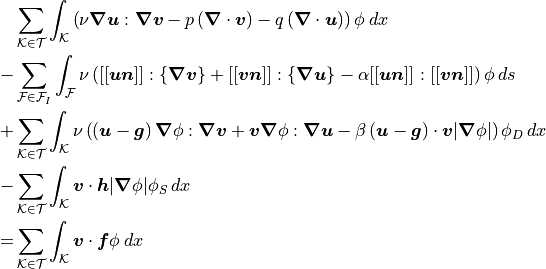## Incompressible Navier-Stokes Equations

The incompressible Navier-Stokes equations take the following form on a simulation domain. There are two possible types of boundary condition - velocity Dirichlet and normal stress - applied on boundary regionsandrespectively.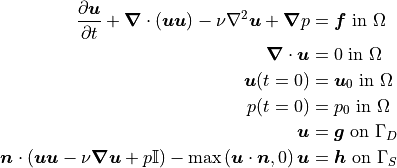whereis the velocity andis the pressure. Furthermore,is the constant kinematic viscosity andis some body force. When Oseen-style linearization is used to linearize the nonlinear convection term, one velocity in said term with be replaced by a known velocity field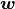(usually the velocity from the previous time step).

The different formulations of the incompressible Navier-Stokes equations finite element weak form are given below. Note that in all casesandare the trial functions for velocity and pressure respectively. In the case of the discontinuous Galerkin method,andrefer to the average and jump operators respectively andis the penalty coefficient. In the case of the diffuse interface method,is the phase field,are masks for different boundary condition regions, andis the Nitsche method penalty parameter. Also note that for the diffuse interface method all integrals become volume integrals over the enclosing simple domain.

### Standard Galerkin Finite Element Formulation

The standard Galerkin finite element method formulation is as follows for Oseen-style linearization: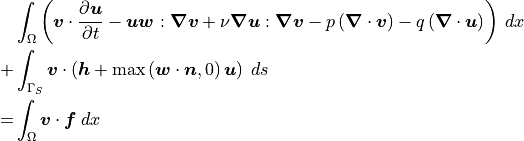and IMEX time discretization: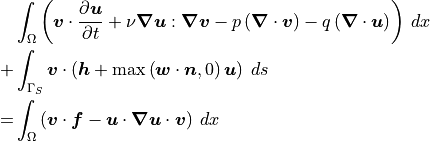In both cases, Dirichlet boundary conditions are imposed strongly by setting values at applicable boundary degrees of freedom.

### Discontinuous Galerkin Formulation

The discontinuous Galerkin formulation is as follows for Oseen-style linearization: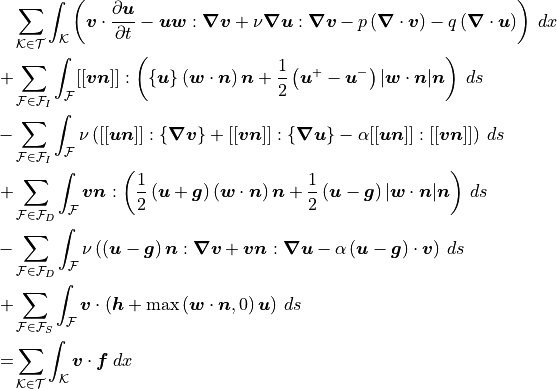and IMEX time discretization: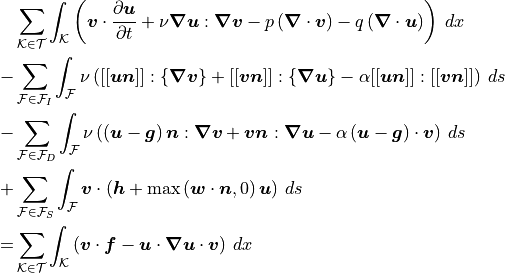In both cases, volume integrals are over individual mesh elementsthen summed over the entire triangulation. Surface integrals are over mesh element facetsand summed over either interior facetsor boundary facetsand.

### Diffuse Interface Formulation

The diffuse interface formulation is as follows for the standard Galerkin finite element method using Oseen-style linearization: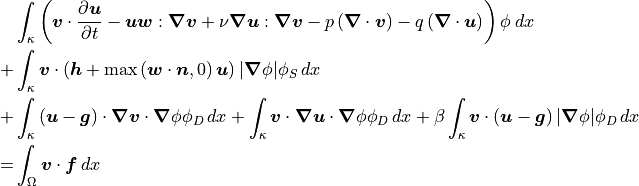and IMEX time discretization: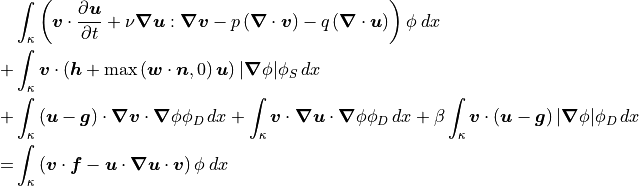The diffuse interface formulation is as follows for the discontinuous Galerkin method using Oseen-style linearization:and IMEX time discretization: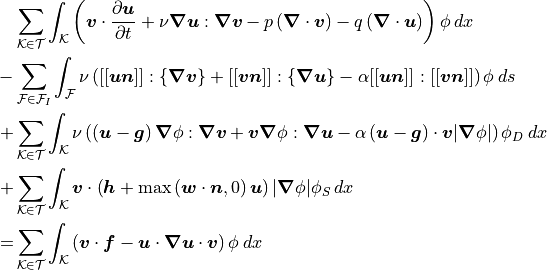## Multi-Component Flow

Note

To be written once discontinuous Galerkin and diffuse interface formulations are available for the multi-component flow model.